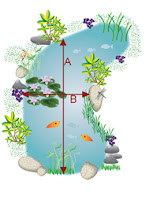## Pages

### Ways to Calculate Pond Volume and SizeHow to Calculate Pond Size
This is the first in a series by South Santee Aquaculture about the various was for calculating the size or volume of a garden pond.

How to Calculate Water Volume

Remember that water volume is calculated much differently based on the shape of the pond.

Oval Pond ------- Length x Width x Depth x 6.7 = Water Volume

Circle Pond ------ Length x Width x Depth x 5.9 = Water Volume

Rectangle Pond -- Length x Width x Depth x 7.5 = Water Volume

So...if the water depth is not the same for the entire pond, estimate the average depth of the water for the pond and use that number for the depth.

Also if you are going to build an oval pond 16 feet long by 11 feet wide by 2 feet deep, the water volume would be:

11 x 16 x 2 x 6.7 = 2358 Gallons

Why is Calculating Pond Size Important?
The calculation of the size and volume of your pond is important to virtually every aspect involved with keeping it well maintained, such as the fish you buy, how to control aquatic life, staying on top of important cleaning tasks and when purchasing fountains or aeration devices.

What about Calculating size of Irregular Shaped Ponds?Irregular shaped ponds
This one is a little bit trickier and we will go into some advanced methods of calculating odd or irregular shaped ponds in the near future.  However, what I will tell you is Irregular shaped garden ponds are calculated using "squares". This is a bit tedious done manually but with the help of South Santee Aquaculture it can be greatly simplified and the results for your garden ponds volume are very accurate when care is taken.  Contact us with questions about your pond.

The system relies upon this basic formula ...
Pond Volume = Surface Area x Average Depth.

The challenge lies in calculating the surface area accurately which is easy if the shape is a square or round say. But for complex shapes an estimate of area has to be made using the "count the squares" technique.

Related Posts of Interest:
Yearly Pond Maintenance Calendar